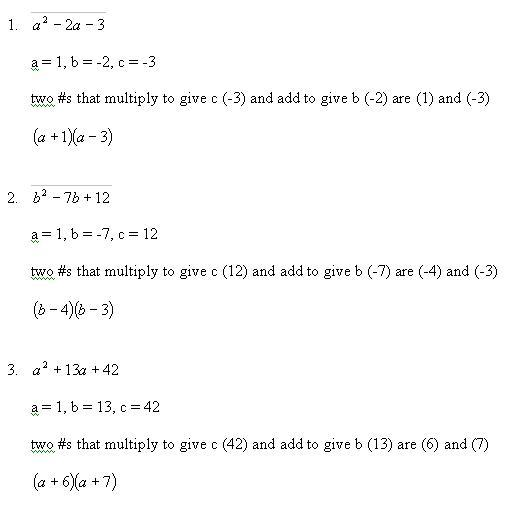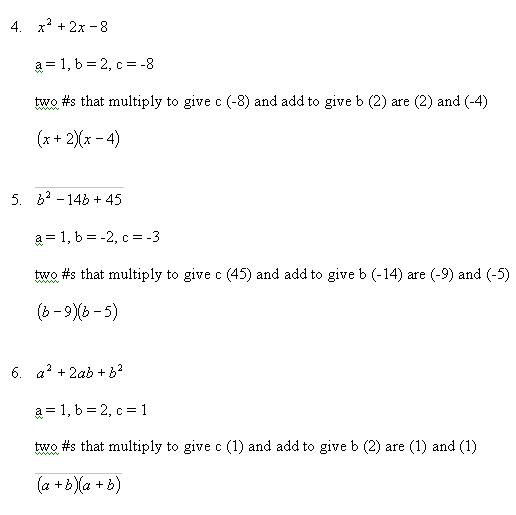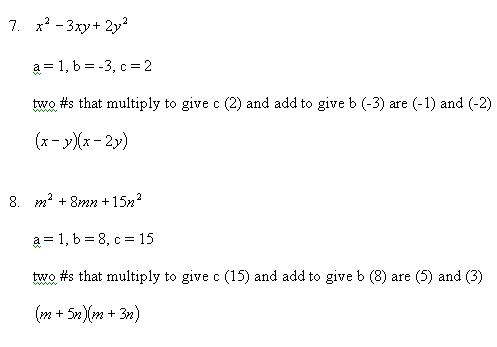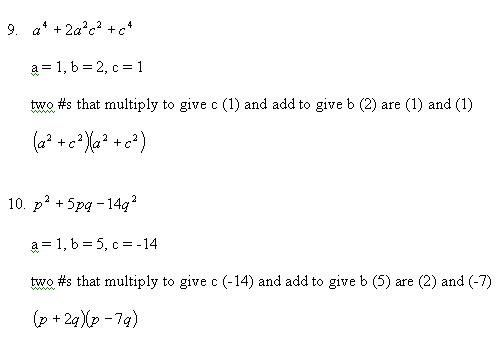HOME

NOTE: There are 2 mistakes on the answer sheet below:

In question #4, the two numbers are 4 and -2, and the answer is      (x+4)(x-2), and in question #10, the two numbers are 7 and -2,         and the answer is (p+7q)(p-2q).